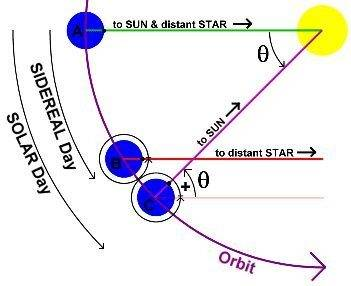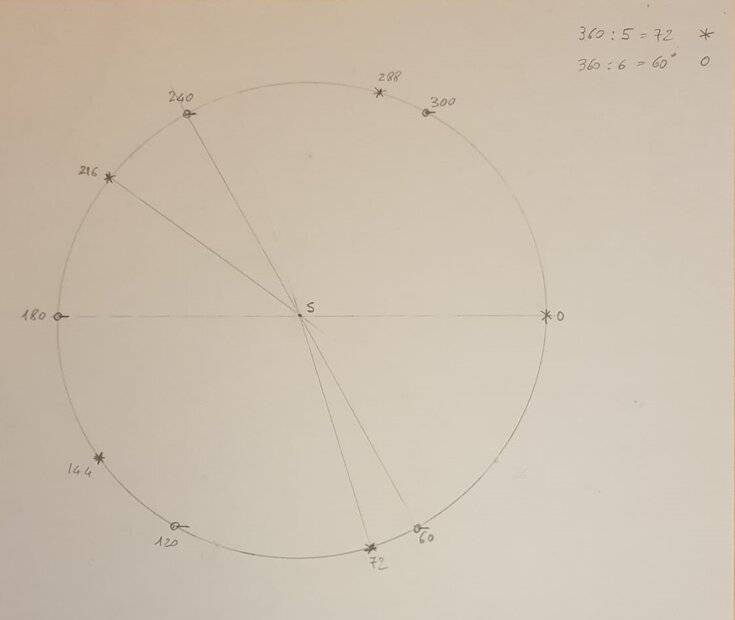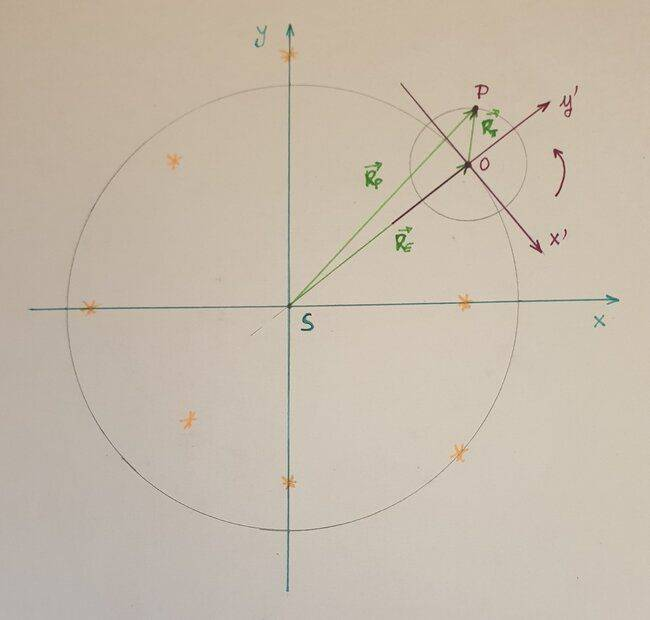# About the relation between number of sidereal and solar days

• I
Summary:
How to work out the formula relating the number of sidereal vs the number of solar days for a generic planet orbit.
Hi all,

I've a doubt about the following formula for the number of sidereal vs solar days for a generic planet orbit (e.g. the Earth's orbit around the Sun):

$$N_{sid} = N_{sol} + 1$$
Section 1.5 of the book "Foundation of Astrophysics" - B. Ryden shows how to calculate the above equation starting from the angular velocity ##\vec \omega_{sid}## of the Earth's rotation in the non-rotating sidereal frame where "fixed starts" are at rest and the angular velocity ##\vec \omega_{E}## of the Earth's orbital motion in the same sidereal frame.

$$\vec \omega_{sid} (t) = \vec \omega_{sol} (t) + \vec \omega_{E} (t)$$
##\vec \omega_{sol} (t)## is the angular velocity of the Earth's rotation in a reference frame that co-rotates with the Earth-Sun line.

As far as I can understand ##\vec \omega_{sid} (t)## actually represents the whole Earth's angular velocity that include all the 'rotation components', not just the angular velocity of the Earth spinning around its North-South axis.

Is that correct ? Thanks you.

Last edited:

Staff Emeritus
Since the planet gets one rotation "for free" when it orbits, don't you just add one? 365 solar days is 366 sidereal days.

•sophiecentaur, phyzguy and Orodruin
Since the planet gets one rotation "for free" when it orbits, don't you just add one? 365 solar days is 366 sidereal days.
Do you mean it gets "for free" a rotation around its North-South axis when a revolution around the Sun is completed ?

phyzguy
Think of the case where the planet does not rotate at all relative to the distant stars. The sun will still rise and set once during the course of a year.

•Think of the case where the planet does not rotate at all relative to the distant stars. The sun will still rise and set once during the course of a year.
So in the course of a year there will be 1 solar day and zero sidereal day, don't you ?

phyzguy
So in the course of a year there will be 1 solar day and zero sidereal day, don't you ?
Yes, exactly.

Sorry, but the relation should be in the other way: ##N_{sid}=N_{sol} + 1##

phyzguy
Sorry, but the relation should be in the other way: ##N_{sid}=N_{sol} + 1##
It depends on the relative direction of the two rotations. In the case of the Earth, the rotation about the axis and the revolution about the sun are in the same direction, so Nsid = Nsol + 1. In the hypothetical case of a planet which does not rotate relative to the distant stars, the rotation about the axis in the frame of fixed planet-sun line is opposite to the rotation about the sun, so Nsid = Nsol - 1.

In the hypothetical case of a planet which does not rotate relative to the distant stars, the rotation about the axis in the frame of fixed planet-sun line is opposite to the rotation about the sun, so Nsid = Nsol - 1.
ok got it.

Sorry for the basic question about the planet as the Earth (surely it is not a level I question)

As in the picture, start with the sun at noon at the reference meridian. From the beginning of the second sidereal day the Earth must rotate further around its axis to sweep an angle of about 1 degree (0.9856 degree) in order to re-align the reference meridian with the sun (this basically amounts to 3m 56s as the difference in duration between a solar day and a sidereal day).What about the second solar day ? This time -- starting from the beginning of the third sidereal day -- the angle the Earth's rotation has to sweep is actually the double of the previous one (2x0.9856 = 1,9712 degree).

If the above is correct, does the difference between solar vs sidereal day remain constant over the year (3m 56s) ?

Thanks.

Last edited:
phyzguy
ok got it.

Sorry for the basic question about the planet as the Earth (surely it is not a level I question)

As in the picture, start with the sun at noon at the reference meridian. From the beginning of the second sidereal day the Earth must rotate further around its axis to sweep an angle of about 1 degree (0.9856 degree) in order to re-align the reference meridian with the sun (this basically amounts to 3m 56s as the difference in duration between a solar day and a sidereal day).

View attachment 289442

What about the second solar day ? This time -- starting from the beginning of the third sidereal day -- the angle the Earth's rotation has to sweep is actually the double of the previous one (2x0.9856 = 1,9712 degree).

If the above is correct, does the difference between solar vs sidereal day remain constant over the year (3m 56s) ?

Thanks.
Your statement for the first day is correct.

The second day is the same as the first. You start from solar noon, not from the beginning of the sidereal day, so it takes an addition 3m 56s to come back to solar noon. If you start from the same sidereal time each day, it is true that the difference between solar time and sidereal time accumulates as you move through the year. On the autumnal equinox, the difference is zero, and six months later on the vernal equinox, the difference is 12 hours.

The difference between the solar day and the sidereal day is not exactly the same through the year. The time for the Earth to rotate on its axis stays the same through the year to high accuracy. But the rate of motion of the Earth around the orbit changes because sometimes the Earth is slightly closer to the sun than others. When it is closer to the sun, it moves faster. The difference through the year is summarized as the Equation of Time. The 3m 56s is the average difference through the year.

•hussein
ok, so starting from the beginning (e.g. the autumnal equinox) we have:• the time difference between the end of the first sidereal day (position B) and the next solar noon (position C - end of the first solar day) is 3m 56s (235.9 s)
• the time difference between the end of the second sidereal day and the next solar noon (second solar noon - end of the second solar day) should be 2*235.9 = 471.8 s
• the time difference between the end of the third sidereal day and the next solar noon (third solar noon - end of the third solar day) should be 3*235.9 = 707.7 s
and so on....thus at the end of the 365 day (actually 365,2564) the time difference should be 365,2564*235.9 = 86163.98 s which amounts to a sidereal day.

Hence in a sidereal year the number of sidereal days is one plus the number of solar days.

Does it make sense ? Thanks.

phyzguy
Hence in a sidereal year the number of sidereal days is one plus the number of solar days.

Does it make sense ? Thanks.
Yes! I think you've got it.

So the stars in the night sky should rise at different solar time throughout the year, I think.

It should be about 4 minutes earlier each night, hence a whole hour earlier in 15 days and two hours earlier in 30 days and so on up to 24 hours, right ?

Last edited:
phyzguy
Correct. That's why we see different constellations at different times of the year. You might try downloading a copy of Stellarium. It's free, and you can watch how these things change through the year. It's at:
https://stellarium.org/In this picture '*' are the solar noons and 'o' are the "fixed star" noons (i.e. the end/start of a solar day vs the end/start of a sidereal day respectively). As you can see in one sidereal year there are 6 sidereal days and 5 solar days.

Is that correct ? Thank you.

The observer (you) is the small red stick figure.
Thank you, it is really appreciated. I take it as in the animated square on the right (the observer's visible celestial semisphere), the center is the observer's zenith and the apparent motion of the sun and stars occours orthogonal to the observer's upper celestial meridiane (i.e. the part of celestial meridian above the observer's horizon).

Last edited:
phyzguy
Thank you, it is really appreciated. I take it as in the animated square on the right (the observer's visible celestial semisphere), the center is the observer's zenith and the apparent motion of the sun and stars occours orthogonal to the observer's upper celestial meridiane (i.e. the part of celestial meridian above the observer's horizon).
Yes, exactly.

ok, good. However I still have not a clear understanding of the formula found in the B. Ryden book (see post#1).

In the (sidereal) inertial rest frame of the fixed stars centered on the sun, a point on the Earth surface has a composite motion due to the spinning around its axis and the revolution around the Sun. The book seems to describe such motion in that inertial sidereal frame as having an angular velocity ##\omega_{sid}##.

Does it actually refer to that Earth 'composite' motion or just to Earth' s rotation around its axis w.r.t. the fixed stars ?

phyzguy
I'm not sure what you don't understand. In your OP, $\omega_{sid}$ is the angular velocity of the Earth relative to the fixed stars. It is constant through the year, to a high level of accuracy. $\omega_{E}$ is the angular velocity of the Earth around the sun. It varies through the year as the Earth speeds up and slows down in its orbit. $\omega_{sol}$ is the angular velocity of the sun moving around the Earth as it rises and sets each day. It also varies through the year as the Earth speeds up and slows down in its orbit. They are related by the equation you posted in the OP. Which one is a "composite" of the other two is a matter of definition. I would tend to view $\omega_{sol}$ as a "composite" of the other two, but you can look at it however you want to.

In your OP, $\omega_{sid}$ is the angular velocity of the Earth relative to the fixed stars. It is constant through the year, to a high level of accuracy.
How is defined the angular velocity of the Earth relative to the fixed stars ? Which is the center of rotation w.r.t. calculate the derivative of the angle swept from a point on the Earth surface ?

Just to be clear: in the animated square on the left in your previous post take an inertial sidereal frame having the center on the sun and in which stars are at rest. The observer's head (a point on the Earth surface) has actually a complicated motion in that reference frame. Which is its angular velocity ##\omega_{sid}## in this case ?

Last edited:
phyzguy
The center of rotation for $\omega_{sid}$ is the Earth's axis. It's true that the motion of the Earth around the sun makes the motion complicated, but the stars are so far away that that motion is negligible. For example, to measure $\omega_{sid}$, I can just start a stopwatch when a distant star crosses the meridian, and then stop the stopwatch when it crosses the meridian again a (sidereal) day later.

Bandersnatch
Just a note here: this problem can be expressed equivalently with clock hands instead of celestial bodies. All the expressions work the same, while it might be easier to see what's going on.
For reference: Earth is in the centre of the clock, marks on the clock face are distant stars, the minute hand represents Earth's rotation (e.g. position of some meridian on its surface), and the hour hand represents yearly movement of the Sun (i.e. the direction towards the Sun).
The hands meet 11 times (solar days) per single rotation of the hour hand (a year), during which the minute hand rotates 12 times (sidereal days) w/r to the clock face (distant stars). The 'loss' of one meeting of the hands every 12 hours is the same as losing one day per year when using the solar instead of the sidereal count.
The angular velocities are that of each hand w/r to the clock face (minute=##\omega_{sid}##, hour=##\omega_{E}##), and of one hand w/r to the other (##\omega_{sol}##).

•cianfa72 and phyzguy
The center of rotation for $\omega_{sid}$ is the Earth's axis. It's true that the motion of the Earth around the sun makes the motion complicated, but the stars are so far away that that motion is negligible.
To help my understanding I made this diagramThe are basically two reference frames involved: the first one (S,x,y) having the center in the sun (S) in which distant stars are at rest and the second one (O,x',y') co-moving with the Earth-Sun line during the sidereal year.

##\vec R_P (t)## is the position vector of the point P (a given point P on the Earth surface) in the (S,x,y) frame, ##\vec R_E (t)## is the position vector of the Earth's center in the same (S,x,y) frame and ##\vec R_T (t)## is the point P position vector in the (O,x',y') frame.

Now ##\vec R_T (t) = \vec R_P (t) - \vec R_E (t)## and in the (O,x',y') frame the point P rotates around the origin O with angular velocity ##\omega_{sol}##.

As we said, the motion of P in the (S,x,y) frame is complicated due to the revolution of the Earth around the sun, so I believe in the (S,x,y) frame we cannot actually claim the point P just rotates with a given angular velocity - since after a turn of the Earth around itself the point P does not return on the same point in the (S,x,y) frame.

So basically what you are saying is that if we did the exact calculation -- i.e. the derivative of ##\vec R_T (t)## in the (O,x',y') frame -- we would find that the angular velocity ##\omega_{sol}## of the point P in the (O,x',y') frame should be exactly equal to:
$$\omega_{sol} = \omega_{sid} - \omega_{E}$$
##\omega_E## is the angular velocity of the center of the Earth (point O) in the (S,x,y) frame.

Does it make sense ? Thank you.

Last edited:
Just a note here: this problem can be expressed equivalently with clock hands instead of celestial bodies. All the expressions work the same, while it might be easier to see what's going on.
For reference: Earth is in the centre of the clock, marks on the clock face are distant stars, the minute hand represents Earth's rotation (e.g. position of some meridian on its surface), and the hour hand represents yearly movement of the Sun (i.e. the direction towards the Sun).
The hands meet 11 times (solar days) per single rotation of the hour hand (a year), during which the minute hand rotates 12 times (sidereal days) w/r to the clock face (distant stars). The 'loss' of one meeting of the hands every 12 hours is the same as losing one day per year when using the solar instead of the sidereal count.
The angular velocities are that of each hand w/r to the clock face (minute=##\omega_{sid}##, hour=##\omega_{E}##), and of one hand w/r to the other (##\omega_{sol}##).
Instead of hour and minute hands, you could have a clock which has a hour hand and a month hand. This way, the full circle of clock face is divided into 12 months and 24 hours - both neatly divisible by 12 (which itself is divisible by 4).

•cianfa72